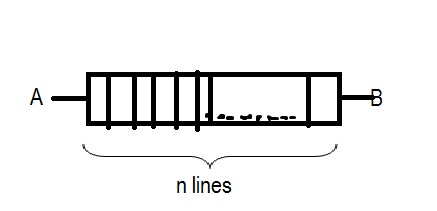# Want to know the methodQ1) In how many ways a triangle can be formed by choosing numbers from the set $\{1,2,3...,10\}$ .

Q2) I dont know the question exactly, But it says six ants are on the edge of a regular octahedron such that no two are on the same edge.They start moving on the adjacent edges, find the probability that no two ants arrive on the same vertex.

Q3) the figure is above, in how many ways can $A$ reach $B$?

Q4) Number of integral pairs $(x,y)$ satisfying $a+ib=(a+ib)^{2002}$ where $i=\sqrt{-1}$Note by Tanishq Varshney
6 years, 1 month ago

This discussion board is a place to discuss our Daily Challenges and the math and science related to those challenges. Explanations are more than just a solution — they should explain the steps and thinking strategies that you used to obtain the solution. Comments should further the discussion of math and science.

When posting on Brilliant:

• Use the emojis to react to an explanation, whether you're congratulating a job well done , or just really confused .
• Ask specific questions about the challenge or the steps in somebody's explanation. Well-posed questions can add a lot to the discussion, but posting "I don't understand!" doesn't help anyone.
• Try to contribute something new to the discussion, whether it is an extension, generalization or other idea related to the challenge.
• Stay on topic — we're all here to learn more about math and science, not to hear about your favorite get-rich-quick scheme or current world events.

MarkdownAppears as
*italics* or _italics_ italics
**bold** or __bold__ bold
- bulleted- list
• bulleted
• list
1. numbered2. list
1. numbered
2. list
Note: you must add a full line of space before and after lists for them to show up correctly
paragraph 1paragraph 2

paragraph 1

paragraph 2

[example link](https://brilliant.org)example link
> This is a quote
This is a quote
    # I indented these lines
# 4 spaces, and now they show
# up as a code block.

print "hello world"
# I indented these lines
# 4 spaces, and now they show
# up as a code block.

print "hello world"
MathAppears as
Remember to wrap math in $$ ... $$ or $ ... $ to ensure proper formatting.
2 \times 3 $2 \times 3$
2^{34} $2^{34}$
a_{i-1} $a_{i-1}$
\frac{2}{3} $\frac{2}{3}$
\sqrt{2} $\sqrt{2}$
\sum_{i=1}^3 $\sum_{i=1}^3$
\sin \theta $\sin \theta$
\boxed{123} $\boxed{123}$

## Comments

Sort by:

Top Newest

These All are questions from IPU CET 2015 which was held on 17th May,2015 @Tanishq Varshney , I too want to know the answers .For Q2 i think there's already a probem (similar) on b'ant, i just don't recall it correctly

- 6 years, 1 month ago

Log in to reply

add me up on whatsapp with +2348029770297

- 6 years, 1 month ago

Log in to reply

Who in the blue hell, r u jabroni , and i feel that no one is lunatic enough to add u or contact u 😝 becoz u r a complete stranger to me

- 6 years, 1 month ago

Log in to reply

Q4) It is easy to see that $|a+ib|=1 or 0$. Therefore, the answer will consist of all the $2001^{th}$ roots of unity, as well as $0+0i$.

- 6 years, 1 month ago

Log in to reply

Q3. This is a binary situation, either up or down path hence total ways are $\displaystyle {2} ^ {n - 1}.$

- 6 years, 1 month ago

Log in to reply

Sir, Do you mind explaining? I can't understand the formula : $2^{n-1}$

- 6 years, 1 month ago

Log in to reply

To reach B , the nth line must be compulsorily taken and hence the nth line has only one choice. But in the first (n-1) lines one can take the upper path or the lower path, hence each of these (n-1) lines has 2 possibilities. Thus by product rule , total ways $= 2^{n-1} \times 1 = 2^{n-1}$ ways. Try a similar problem here.

- 6 years, 1 month ago

Log in to reply

- 6 years, 1 month ago

Log in to reply

Try playing with small cases for motivation and ease. Then see if you can arrive at a general idea. I say, start with a set $A = \{1,2,3,4\}$.

- 6 years, 1 month ago

Log in to reply

Dude, the triangles question. I think you can only do it by counting.

Take three cases: equilateral, isoceles and scalene.

Equilateral is simple.

Isoceles : Let the sides be $a$ and $b$ and let $a$ be the side which is equal. Then probably use Triangle Inequality.

Scalene Take two cases right triangles and normal triangles. Again triangle inequality must do the trick.

My method is very long. There must be a shorter way. Hope there is.

- 6 years, 1 month ago

Log in to reply

- 6 years, 1 month ago

Log in to reply

sorry guys i don't have their answers

- 6 years, 1 month ago

Log in to reply

×

Problem Loading...

Note Loading...

Set Loading...